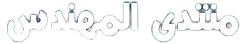# مساعدة عاجلة

السلام عليكم
اذا ممكن مساعدة في حل هاي الاسئلة
Q2: The steady state temperature distribution in a one-dimensional wall of thermal conductivity 50 W/m.K and thickness 60 mm is observed to be T(C) = a + bx2, where a = 200C, b = -2000C/m2 and x is in meters.

1. What is the heat generation rate in the wall?
2. Determine the heat fluxes at the two wall faces. In what manner are these heat fluxes related to the heat generation rate?

Q3: Uniform internal heat generation at 5 X 107 W/m3 is occurring in a cylindrical nuclear reactor fuel rod of 50-mm diameter and under steady-state conditions the temperature distribution is of the form , where T in C and r is in meters, while a = 800C and b = -4.167 X 105 C/W. the fuel rod properties are k = 30 W/m. K,  = 1100 kg/m3 and cp = 800 J/kg. K. What is the rate of heat transfer per unit length of the rod at r = 0 (the centerline) and at r = 25 mm (the surface)?